NABARD Quantitative Aptitude Practice: 1

# NABARD Quantitative Aptitude Practice: 1

Test Description

## 30 Questions MCQ Test NABARD Assistant Manager Grade B Mock Test Series | NABARD Quantitative Aptitude Practice: 1

NABARD Quantitative Aptitude Practice: 1 for Banking Exams 2022 is part of NABARD Assistant Manager Grade B Mock Test Series preparation. The NABARD Quantitative Aptitude Practice: 1 questions and answers have been prepared according to the Banking Exams exam syllabus.The NABARD Quantitative Aptitude Practice: 1 MCQs are made for Banking Exams 2022 Exam. Find important definitions, questions, notes, meanings, examples, exercises, MCQs and online tests for NABARD Quantitative Aptitude Practice: 1 below.
Solutions of NABARD Quantitative Aptitude Practice: 1 questions in English are available as part of our NABARD Assistant Manager Grade B Mock Test Series for Banking Exams & NABARD Quantitative Aptitude Practice: 1 solutions in Hindi for NABARD Assistant Manager Grade B Mock Test Series course. Download more important topics, notes, lectures and mock test series for Banking Exams Exam by signing up for free. Attempt NABARD Quantitative Aptitude Practice: 1 | 30 questions in 25 minutes | Mock test for Banking Exams preparation | Free important questions MCQ to study NABARD Assistant Manager Grade B Mock Test Series for Banking Exams Exam | Download free PDF with solutions
 1 Crore+ students have signed up on EduRev. Have you?
NABARD Quantitative Aptitude Practice: 1 - Question 1

### In each of these questions two equations (I) and (II) are given. You have to solve both the equations and give answer. I. 2x2 - 21x + 54 = 0 II. y2 - 14y + 49 = 0

Detailed Solution for NABARD Quantitative Aptitude Practice: 1 - Question 1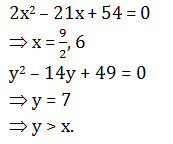NABARD Quantitative Aptitude Practice: 1 - Question 2

### In each of these questions two equations (I) and (II) are given. You have to solve both the equations and give answer. I. x2 - 19x + 70 = 0 II. 2y2 - 17y + 35 = 0

Detailed Solution for NABARD Quantitative Aptitude Practice: 1 - Question 2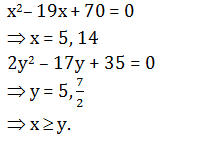NABARD Quantitative Aptitude Practice: 1 - Question 3

### In each of these questions two equations (I) and (II) are given. You have to solve both the equations and give answer. I. 3x2 + 5x - 8 = 0 II. y2 - 4y + 3 = 0

Detailed Solution for NABARD Quantitative Aptitude Practice: 1 - Question 3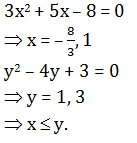NABARD Quantitative Aptitude Practice: 1 - Question 4

In each of these questions two equations (I) and (II) are given. You have to solve both the equations and give answer.

I. 3x2 + 11x + 8 = 0
II. 3y2 + 20y + 32 = 0

Detailed Solution for NABARD Quantitative Aptitude Practice: 1 - Question 4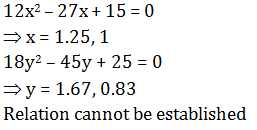NABARD Quantitative Aptitude Practice: 1 - Question 5

In the following number series, a wrong number is given. Find out that wrong number.

2, 11, 38, 197, 1172, 8227, 65806

Detailed Solution for NABARD Quantitative Aptitude Practice: 1 - Question 5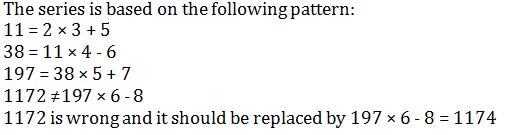NABARD Quantitative Aptitude Practice: 1 - Question 6

In each of these questions two equations (I) and (II) are given. You have to solve both the equations and give answer.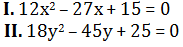Detailed Solution for NABARD Quantitative Aptitude Practice: 1 - Question 6NABARD Quantitative Aptitude Practice: 1 - Question 7

In the following number series, a wrong number is given. Find out that wrong number.

16, 19, 21, 30, 46, 71, 107

Detailed Solution for NABARD Quantitative Aptitude Practice: 1 - Question 7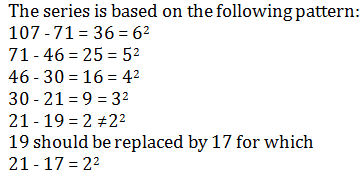NABARD Quantitative Aptitude Practice: 1 - Question 8

In the following number series, a wrong number is given. Find out that wrong number.

7.5, 47.5, 87.5, 157.5, 247.5, 357.5, 487.5

Detailed Solution for NABARD Quantitative Aptitude Practice: 1 - Question 8

The given number series is based on the following pattern :
487.5 - 357.5 = 130
357.5 - 247.5 = 110
247.5 - 157.5 = 90
157.5 - 87.5 = 70
87.5 - 47.5 = 40
87.5 - 37.5 = 50
37.5 - 7.5 = 30
Clearly, 47.5 is the wrong number. It should be replaced by 37.5.

NABARD Quantitative Aptitude Practice: 1 - Question 9

In the following number series, a wrong number is given. Find out that wrong number.

13, 16, 21, 27, 39, 52, 69

Detailed Solution for NABARD Quantitative Aptitude Practice: 1 - Question 9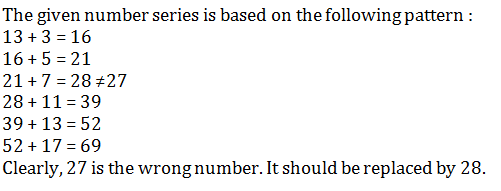NABARD Quantitative Aptitude Practice: 1 - Question 10

In the following number series, a wrong number is given. Find out that wrong number.

1500, 1581, 1664, 1749, 1833, 1925, 2016

Detailed Solution for NABARD Quantitative Aptitude Practice: 1 - Question 10

The pattern is:+81,+83,+85,+87,+89,+91Clearly 1833is the wrong number.It should be replaced by 1836

NABARD Quantitative Aptitude Practice: 1 - Question 11

Study the following graph and answer the questions given below.
Runs scored by three different teams in five different cricket matches.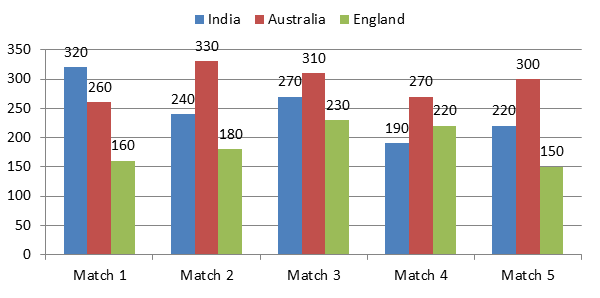Q. The total runs scored by India and Australia in Match 4 together is approximately what percentage of the total runs scored by England in all the five matches together?

Detailed Solution for NABARD Quantitative Aptitude Practice: 1 - Question 11

Total runs scored by India and Australia in Match 4 together = 270 + 190 = 460
Total runs scored by England in all the five matches together= 160 + 180+ 230+ 220 + 150 = 940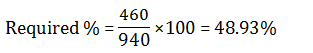NABARD Quantitative Aptitude Practice: 1 - Question 12

Study the following graph and answer the questions given below.
Runs scored by three different teams in five different cricket matches.Q. In which match is the difference between the runs scored by Australia and England the second lowest?

Detailed Solution for NABARD Quantitative Aptitude Practice: 1 - Question 12

Difference between Australia and England in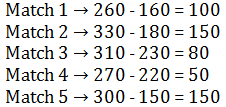The second lowest difference of runs scored was in Match 3.

NABARD Quantitative Aptitude Practice: 1 - Question 13

Study the following graph and answer the questions given below.
Runs scored by three different teams in five different cricket matches.Q. In which match are the total runs scored by India and England together the third highest/ lowest?

Detailed Solution for NABARD Quantitative Aptitude Practice: 1 - Question 13

Total runs scored by India and England in Match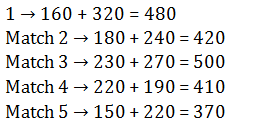Hence the third highest/lowest was scored in Match 2.

NABARD Quantitative Aptitude Practice: 1 - Question 14

Study the following graph and answer the questions given below.
Runs scored by three different teams in five different cricket matches.Q. What is the ratio of the runs scored by India in Match 5, Australia in Match 1 and England in Match 2?

Detailed Solution for NABARD Quantitative Aptitude Practice: 1 - Question 14

India scored in Match 5 = 220
England scored in Match 2 = 180
Australia scored in Match 1 = 260
Ratio of India : Australia : England
220 : 260 : 180
11 : 13 : 9

NABARD Quantitative Aptitude Practice: 1 - Question 15

Study the following graph and answer the questions given below.
Runs scored by three different teams in five different cricket matches.Q What is the average runs scored by all the three teams in Match 3 together?

Detailed Solution for NABARD Quantitative Aptitude Practice: 1 - Question 15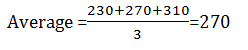NABARD Quantitative Aptitude Practice: 1 - Question 16

What should come in place of question mark (?) in the following equations?

23% of 460 × 35% of 270 × ? % of 172 = 429918.3

Detailed Solution for NABARD Quantitative Aptitude Practice: 1 - Question 16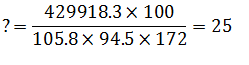NABARD Quantitative Aptitude Practice: 1 - Question 17

What should come in place of question mark (?) in the following equations?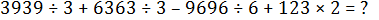Detailed Solution for NABARD Quantitative Aptitude Practice: 1 - Question 17

? = 1313 + 2121 – 1616 + 246 = 2064

NABARD Quantitative Aptitude Practice: 1 - Question 18

What should come in place of question mark (?) in the following equations?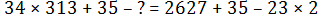Detailed Solution for NABARD Quantitative Aptitude Practice: 1 - Question 18

?=34×313+35-2627-35+46=8061

NABARD Quantitative Aptitude Practice: 1 - Question 19

What should come in place of question mark (?) in the following equations?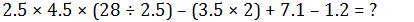Detailed Solution for NABARD Quantitative Aptitude Practice: 1 - Question 19

?=2.5×4.5×11.2-7+7.1-1.2=124.9

NABARD Quantitative Aptitude Practice: 1 - Question 20

What should come in place of question mark (?) in the following equations?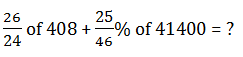Detailed Solution for NABARD Quantitative Aptitude Practice: 1 - Question 20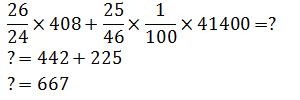NABARD Quantitative Aptitude Practice: 1 - Question 21

A radio dealer sold a radio at a loss of 2.5%. Had he sold it for Rs. 100 more. He would have gained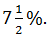In order to gain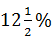he should sell it for

Detailed Solution for NABARD Quantitative Aptitude Practice: 1 - Question 21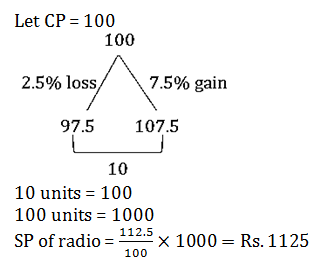NABARD Quantitative Aptitude Practice: 1 - Question 22

One trader calculates the percentage of profit on the buying price and another calculates on the selling price. When their selling prices are the same, then the difference of their actual profits is Rs. 85 and both claim to have made 20% profit, what is the selling price of each?

Detailed Solution for NABARD Quantitative Aptitude Practice: 1 - Question 22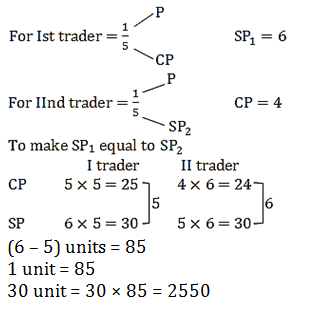NABARD Quantitative Aptitude Practice: 1 - Question 23

A person invests Rs. 12,000 as fixed deposit at a bank at the rate of 10% per annum simple interest. But due to some pressing needs he has to withdraw the entire money after 3 years, for which the bank allowed him a lower rate of interest. If he gets Rs. 3320 less than what he would have got at the end of 5 years, the rate of interest allowed by the bank is:

Detailed Solution for NABARD Quantitative Aptitude Practice: 1 - Question 23

Principal = Rs. 12000Rate % = 10%Interest would have been received by person in 5 years Interest received by the person after 3 years = Rs(6000 – 3320) = Rs 2680.By using formula,Rate %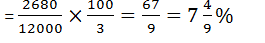NABARD Quantitative Aptitude Practice: 1 - Question 24

On a certain sum of money, the difference between the compound interest for a year, payable half-yearly, and the simple interest for a year is Rs. 180. If the rate of interest in both the cases is 10%, then the sum is:

Detailed Solution for NABARD Quantitative Aptitude Practice: 1 - Question 24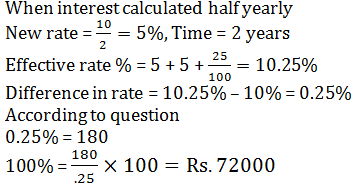NABARD Quantitative Aptitude Practice: 1 - Question 25

Nikita bought 30 kg of wheat at the rate of Rs. 9.50 per kg and 40 kg of wheat at the rate of Rs.8.50 per kg and mixed them. She sold the mixture at the rate of Rs. 8.90 per kg. Her total profit or loss in the transaction was

Detailed Solution for NABARD Quantitative Aptitude Practice: 1 - Question 25

C.P of wheat = 30 × 9.5 + 40 × 8.5
= 285 + 340 = Rs. 625
SP of wheat = 70 × 8.9 = Rs. 623
Loss = CP – SP = 625 – 623 = Rs. 2 Loss

NABARD Quantitative Aptitude Practice: 1 - Question 26

200 litres of a mixture contains milk and water in the ratio 17 : 3. After the addition of some more milk to it, the ratio of milk to water in the resulting mixture becomes 7 : 1. The quantity of milk added to it was

Detailed Solution for NABARD Quantitative Aptitude Practice: 1 - Question 26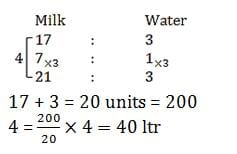NABARD Quantitative Aptitude Practice: 1 - Question 27

A can do a piece of work in 20 days and B in 30 days. They work together for 7 days and then both leave the work. Then C alone finishes the remaining work in 10 days. In how many days will C finish the full work?

Detailed Solution for NABARD Quantitative Aptitude Practice: 1 - Question 27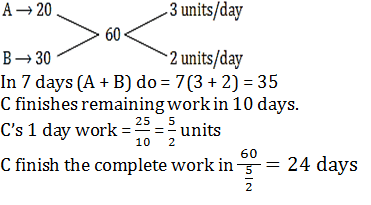NABARD Quantitative Aptitude Practice: 1 - Question 28

A, B, C walk 1 km in 5 minutes 8 minutes and 10 minutes respectively, C starts walking from a point, at a certain time, B starts from the same point 1 minutes later and A starts from the same point 2 minutes later than C. then A meets B and C after.

Detailed Solution for NABARD Quantitative Aptitude Practice: 1 - Question 28

Speed of A, B and C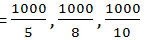= 200m/min, 125m/min, 100m/min
Distance travelled by B and C before A starts = 125, 200 metres
Time taken by A to meet B and C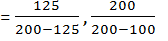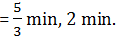NABARD Quantitative Aptitude Practice: 1 - Question 29

A farmer divides his herd of cows among his four sons so that the first son gets one-half the herd, the second son gets one – Fourth, the third son gets one– fifth and the fourth son gets 7 cows. The value of is:

Detailed Solution for NABARD Quantitative Aptitude Practice: 1 - Question 29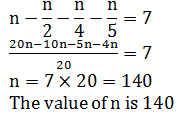NABARD Quantitative Aptitude Practice: 1 - Question 30

The base and altitude of a right angled triangle are 12 cm and 5 cm respectively. The perpendicular distance of its hypotenuse from the opposite vertex is

Detailed Solution for NABARD Quantitative Aptitude Practice: 1 - Question 30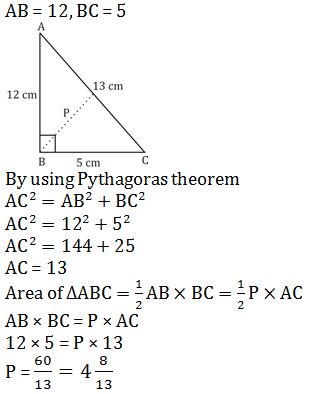## NABARD Assistant Manager Grade B Mock Test Series

1 docs|25 tests
 Use Code STAYHOME200 and get INR 200 additional OFF Use Coupon Code
Information about NABARD Quantitative Aptitude Practice: 1 Page
In this test you can find the Exam questions for NABARD Quantitative Aptitude Practice: 1 solved & explained in the simplest way possible. Besides giving Questions and answers for NABARD Quantitative Aptitude Practice: 1, EduRev gives you an ample number of Online tests for practice

1 docs|25 tests